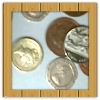#### You may also like### Coin Tossing Games

You and I play a game involving successive throws of a fair coin. Suppose I pick HH and you pick TH. The coin is thrown repeatedly until we see either two heads in a row (I win) or a tail followed by a head (you win). What is the probability that you win?### Win or Lose?

A gambler bets half the money in his pocket on the toss of a coin, winning an equal amount for a head and losing his money if the result is a tail. After 2n plays he has won exactly n times. Has he more money than he started with?A counter is placed in the bottom right hand corner of a grid. You toss a coin and move the star according to the following rules: ... What is the probability that you end up in the top left-hand corner of the grid?

# Same Number!

### Why do this problem?

This problem is one of a set of problems about probability and uncertainty. Intuition can often let us down when working on probability; these problems have been designed to provoke discussions that challenge commonly held misconceptions. Read more in this article.

An important strategy in answering probability questions requires us to consider whether it is easier to work out the probability of an event occurring or NOT occurring, and using the fact that the two probabilities sum to 1. This problem uses this technique.

### Possible approach

For a class of 30, ask everyone to write down a number between $1$ and $225$ without letting anyone see. (If the class size is $N$, ask them to write down a number between $1$ and $(\frac{N}{2})^2$)

Once everyone has chosen, go round the class and ask each person to read out their number, with everyone listening to hear if their number is read out again. On most occasions, there will be duplicates.

"OK, let's have another go, you have $225$ numbers to choose from so let's see if you can manage to make them all different!" (Don't allow collaboration on which numbers to choose!)

The experiment can be repeated a few times, or the interactivity can be used to generate sets of random numbers for a class of 30 to get a sense of how often everyone manages to pick a unique number.

On one occasion, after the first person has read their number, interrupt and ask "What's the probability that the next person has written a different number?", and highlight that it's very likely! Ask a similar question a little further along the line: "What's the probability that the next person has a number that has not been read out so far?" Though the fraction will have changed, it will still be very close to 1, so learners' intuition may be that it is very likely that everyone will choose a different number.

Once everyone has had the chance to be surprised that duplicate numbers happen much more often than not, hand out this worksheet (Word, PDF) and ask the class to discuss the questions and work out their answers with their partner. (You may wish to detach the Extension task from the bottom of the sheet, and hand it out later to those who need it.)

The Extension task is the well-known Birthday Problem; learners could work out the probability of shared birthdays in various class sizes and then use school records to see if the tutor groups in the school have shared birthdays with the expected frequency.

### Key questions

What's the probability (at each stage) that the next person has written a number that has not been read out so far?

What's the probability that the second AND third person (and fourth, and fifth...) have numbers different from the first person and each other?

If I know the probability that every number is different, how can I work out the probability that at least 2 numbers are the same?

### Possible extension

The Birthday Problem provides an interesting extension.

Students might be interested in these articles and related materials on the Understanding Uncertainty website:
Coincidences
What are the chances?
Why coincidences happen

For interested students (or teachers), the "Matching Problems" section of this article by David Spiegelhalter explains the derivation of the $(\frac{N}{2})^2$ formula mentioned above - the maths required to fully understand it is at Stage 5 level.

### Possible support

The problem At Least One... provides an introduction to using tree diagrams and working with mutually exclusive events whose probabilities sum to 1.